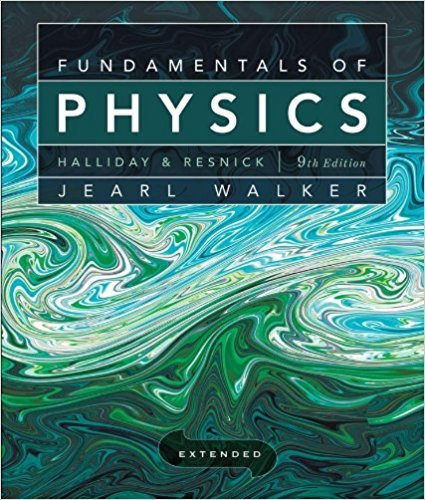×
×

# Solved: Figure 18-53 displays a closed cycle for a gas.ISBN: 9780470469088 189

## Solution for problem 75 Chapter 18

Fundamentals of Physics Extended | 9th Edition

• Textbook Solutions
• 2901 Step-by-step solutions solved by professors and subject experts
• Get 24/7 help from StudySoup virtual teaching assistantsFundamentals of Physics Extended | 9th Edition

4 5 1 384 Reviews
25
2
Problem 75

Figure 18-53 displays a closed cycle for a gas. From e to b, 40 J is transferred from the gas as heat. From b to a, 130 J is transferred from the gas as heat, and the magnitude of the work done by the gas is 801. From a to e, 400 J is transferred to p a '------------v the gas as heat. What is the work Fig. 18-53 75. done by the gas from a to e? (Hint: You need to supply the plus and minus signs for the given data.)

Step-by-Step Solution:
Step 1 of 3
Step 2 of 3

Step 3 of 3

##### ISBN: 9780470469088

This textbook survival guide was created for the textbook: Fundamentals of Physics Extended, edition: 9. Fundamentals of Physics Extended was written by and is associated to the ISBN: 9780470469088. This full solution covers the following key subjects: . This expansive textbook survival guide covers 37 chapters, and 3413 solutions. Since the solution to 75 from 18 chapter was answered, more than 224 students have viewed the full step-by-step answer. The answer to “Figure 18-53 displays a closed cycle for a gas. From e to b, 40 J is transferred from the gas as heat. From b to a, 130 J is transferred from the gas as heat, and the magnitude of the work done by the gas is 801. From a to e, 400 J is transferred to p a '------------v the gas as heat. What is the work Fig. 18-53 75. done by the gas from a to e? (Hint: You need to supply the plus and minus signs for the given data.)” is broken down into a number of easy to follow steps, and 92 words. The full step-by-step solution to problem: 75 from chapter: 18 was answered by , our top Physics solution expert on 12/27/17, 08:15PM.

Unlock Textbook Solution